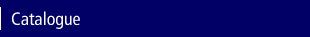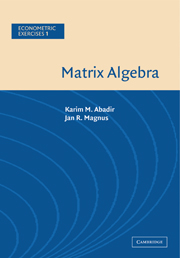Home > Catalogue > Matrix Algebra## Details

• 9 b/w illus.
• Page extent: 466 pages
• Size: 228 x 152 mm
• Weight: 0.74 kg

### Paperback

(ISBN-13: 9780521537469 | ISBN-10: 0521537460)

• There was also a Hardback of this title but it is no longer available | Adobe eBook
• ### For price and ordering options, inspection copy requests, and reading lists please select:

Please note local prices may apply

Matrix Algebra is the first volume of the Econometric Exercises Series. It contains exercises relating to course material in matrix algebra that students are expected to know while enrolled in an (advanced) undergraduate or a postgraduate course in econometrics or statistics. The book contains a comprehensive collection of exercises, all with full answers. But the book is not just a collection of exercises; in fact, it is a textbook, though one that is organized in a completely different manner than the usual textbook. The volume can be used either as a self-contained course in matrix algebra or as a supplementary text.

• Only text that is devoted to solved examples in matrix algebra • Nearly 400 pages of solved problems • Can be used either as a self-contained course in matrix algebra or as a supplementary text

### Contents

Part I. Vectors: 1. Real vectors; 2 Complex vectors; Part II. Matrices: 3. Real matrices; 4. Complex matrices; Part III. Vector Spaces: 5. Complex and real vector spaces; 6. Inner-product space; 7. Hilbert space; Part IV. Rank, Inverse, and Determinant: 8. Rank; 9. Inverse; 10. Determinant; Part V. Partitioned Matrices: 11. Basic results and multiplication relations; 12. Inverses; 13. Determinants; 14. Rank (in)equalities; 15. The sweep operator; Part VI. Systems of Equations: 16. Elementary matrices; 17. Echelon matrices; 18. Gaussian elimination; 19. Homogeneous equations; 20. Nonhomogeneous equations; Part VII. Eigenvalues, Eigenvectors, and Factorizations: 21. Eigenvalues and eigenvectors; 22. Symmetric matrices; 23. Some results for triangular matrices; 24. Schur's decomposition theorem and its consequences; 25. Jordan's decomposition theorem; 26. Jordan chains and generalized eigenvectors; Part VIII. Positive (Semi)Definite and Idempotent Matrices: 27. Positive (semi)definite matrices; 28. Partitioning and positive (semi)definite matrices; 29. Idempotent matrices; Part IX. Matrix Functions: 30. Simple functions; 31. Jordan representation; 32. Matrix-polynomial representation; Part X. Kronecker Product, Vec-Operator, and Moore-Penrose Inverse: 33. The Kronecker product; 34. The vec-operator; 35. The Moore-Penrose inverse; 36. Linear vector and matrix equations; 37. The generalized inverse; Part XI. Patterned Matrices, Commutation and Duplication Matrix: 38. The commutation matrix; 39. The symmetrizer matrix; 40. The vec-operator and the duplication matrix; 41. Linear structures; Part XII. Matrix Inequalities: 42. Cauchy-Schwarz type inequalities; 43. Positive (semi)definite matrix inequalities; 44. Inequalities derived from the Schur complement; 45. Inequalities concerning eigenvalues; Part XIII. Matrix calculus: 46. Basic properties of differentials; 47. Scalar functions; 48. Vector functions; 49. Matrix functions; 50. The inverse; 51. Exponential and logarithm; 52. The determinant; 53. Jacobians; 54. Sensitivity analysis in regression models; 55. The Hessian matrix; 56. Least squares and best linear unbiased estimation; 57. Maximum likelihood estimation; 58. Inequalities and equalities.

### Reviews

'These authors have achieved the remarkable feat of writing a textbook of matrix algebra cunningly concealed as a structured sequence of exercises and worked answers. The book should prove popular with students intent on teaching themselves and with instructors who wish to set challenging and educative exercises. Recommended unequivocally to all parties.' Dr Stephen Pollock, Queen Mary College

'Useful as a text or reference, it is clearly written and very thorough. Besides basic topics, excellent treatment of matrix inequalities, vectorization, and matrix calculus. It belongs on every econometricians's bookshelf.' Professor Peter Schmidt, Michigan State University

'Matrix Algebra can be recommended to teachers and graduate students in all fields of mathematics.' Zentralblatt MATH